SLUSA75B July   2010  – January 2020

PRODUCTION DATA.

1. Features
2. Applications
3. Description
1.     Device Images
4. Revision History
5. Description (continued)
6. Pin Configuration and Functions
7. Specifications
8. Detailed Description
1. 8.1 Overview
2. 8.2 Functional Block Diagram
3. 8.3 Feature Description
4. 8.4 Device Functional Modes
9. Application and Implementation
1. 9.1 Application Information
2. 9.2 Typical Application
1. 9.2.1 Design Requirements
2. 9.2.2 Detailed Design Procedure
3. 9.2.3 Application Curves
10. 10Power Supply Recommendations
11. 11Layout
12. 12Device and Documentation Support
13. 13Mechanical, Packaging, and Orderable Information

• RVA|16
• RVA|16

#### 9.2.2.6 MPPT Temperature Compensation

A typical solar panel comprises of a lot of cells in a series connection, and each cell is a forward-biased p-n junction. So, the open-circuit voltage (VOC) of a solar cell has a temperature coefficient that is similar to a common p-n diode, or about –2 mV/°C. A crystalline solar panel specification always provides both open-circuit voltage VOC and peak power point voltage VMP. The difference between VOC and VMP can be approximated as fixed and temperature-independent, so the temperature coefficient for the peak power point is similar to that of VOC. Normally, panel manufacturers specify the 25°C values for VOC and VMP, and the temperature coefficient for VOC, as shown in Figure 17.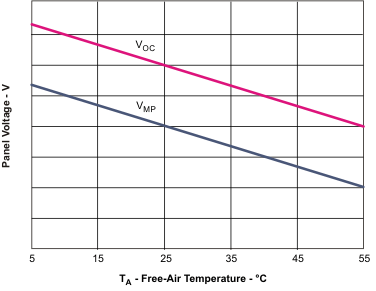Figure 17. Solar Panel Output Voltage Temperature Characteristics

The BQ24650 employs a feedback network to the MPPSET pin to program the input regulation voltage. Because the temperature characteristic for a typical solar panel VMP voltage is almost linear, a simple solution for tracking this characteristic can be implemented by using an LM234 3-terminal current source, which can create an easily programmable, linear temperature dependent current to compensate the negative temperature coefficient of the solar panel output voltage.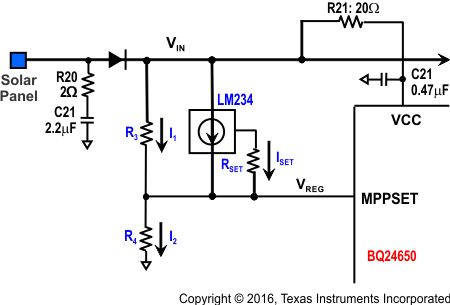Figure 18. Feedback Network

In the circuit shown in Figure 18, for the LM234 temperature sensor,

Equation 24.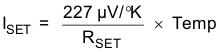Thus,

Equation 25.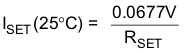The current node equation is Equation 26:

Equation 26.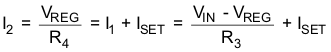To have a zero temperature coefficient on VREG,

Equation 27.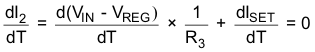Equation 28.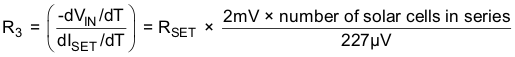Equation 29.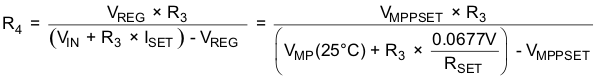For example, given a common 18-cell solar panel that has the following specified characteristics:

Open-circuit voltage (VOC) = 10.3 V
Maximum power voltage (VMP) = 9V
Open-circuit voltage temperature coefficient (VOC) = –38 mV/°C

Applying the following parameters into the equations of R3 and R4:

1. Temperature coefficient for VMP (same as that of VOC) of –38 mV/°C
2. Peak power voltage of 9 V
3. MPPSET regulation voltage of 1.2 V
And choosing RSET = 1000 Ω.

The resistor values are RSET = 1 kΩ, R3 = 167.4 kΩ, and R4=10.6 kΩ. Selecting standard 1% accuracy resistors and RSET = 1 kΩ, R3 = 169 kΩ, and R4=10.7 kΩ.